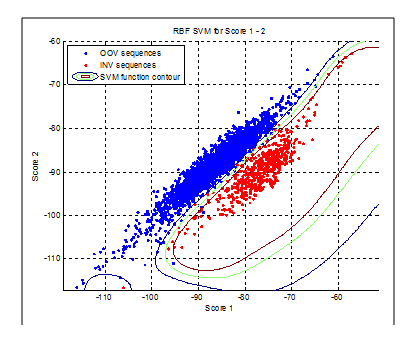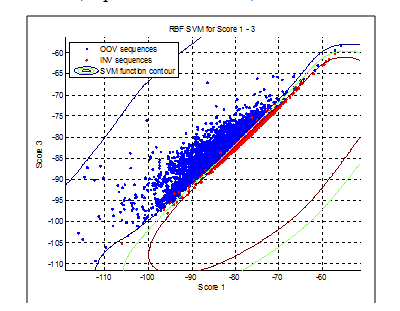# 唤醒系统详解

## Wake-Up-Word system

The concepts of WUW have been most recently expanded in (Këpuska & Klein, 2009). Currently, the system is implemented in C++ as well as Objective C, and provides four major components for achieving the goals of WUW for use in a real-time environment.

1. WUW Front End – This system component is responsible for extracting features from the input audio signal. The current system is capable of extracting Mel-Filtered Cepstral Coefficients (MFCC), Linear Predictive Coding coefficients (LPC), and enhanced MFCC features.

2. Voice Activity Detector (VAD) – A large portion of the input audio signal to the system are non-speech events such as silence or environmental noise. Filtering this information is critical in order to ensure the system is only listening during speech events of interest. Areas of audio that are determined to be speech-related are then forwarded to the later stages of the WUW system.

3. WUW Back End – The Back End performs a complex recognition procedure based on Hidden Markov Models (HMMs). HMMs are continuous densities HMM‘s.

4. SVM Classification - The final system component is responsible for classifying speech signals as In-Vocabulary (INV) or Out-of-Vocabulary (OOV) using Support Vector Machines (SVMs). In the WUW context, the only INV word is the one selected for command and control of the host system. Any other word or sound is classified as OOV.

The following diagram illustrates the top-level workflow of the WUW system: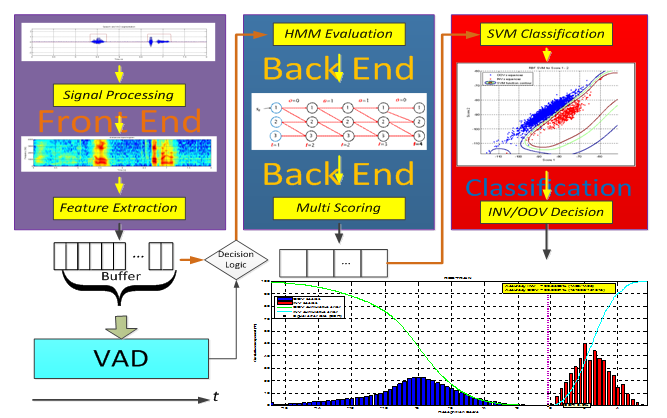### 1. WAKE-UP-WORD FRONT END

The front end is responsible for extracting features out of the input signal. Three sets of features are extracted: Mel-Filtered Cepstral Coefficients (MFCC), LPC (Linear Predictive Coding) smoothed MFCCs, and Enhanced MFCCs.

The following image, Figure 2, shows a waveform superimposed with its VAD segmentation, its spectrogram, and its enhanced spectrogram.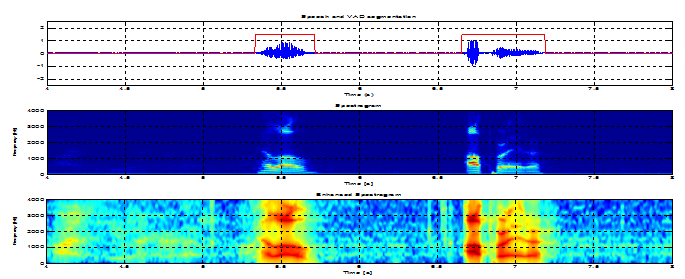The MFCCs are computed using the standard algorithm as presented in the Figure 3: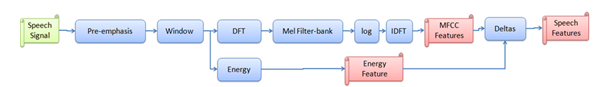Pre-emphasis – This stage is used to amplify energy in the high-frequencies of the input speech signal. This allows information in these regions to be more recognizable during HMM model training and recognition.

Windowing – This stage slices the input signal into discrete time segments. This is done by using window N milliseconds typically 25 ms wide and at offsets of M milliseconds long. A Hamming window is commonly used to prevent edge effects associated with the sharp changes in a Rectangular window. Equation 1 and Figure 5show the equation for the, typiccally 10 ms or 5 msHamming window and its effect when it is applied to a speech signal.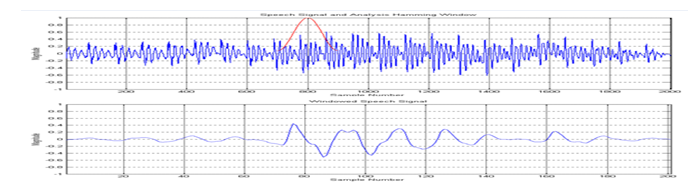Discrete Fourier Transform – DFT is applied to the windowed speech signal, resulting in the magnitude and phase representation of the signal. The log-magnitude of an example speech signal is depicted in Figure 4.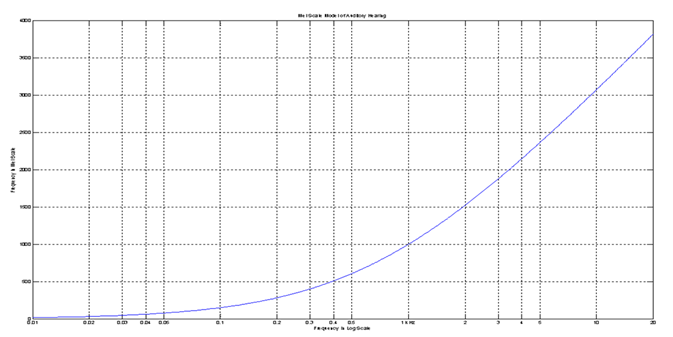Mel Filter Bank – While the resulting spectrum of the DFT contains information in each frequency, human hearing is less sensitive at frequencies above 1000 Hz. This concept also has a direct effect on performance of ASR systems; therefore, the spectrum is warped using a logarithmic Mel scale (see Figure 6. below). A Mel frequency can be computed using equation 3. In order to create this effect on the DFT spectrum, a bank of filters is constructed with filters distributed equally below 1000 Hz and spaced logarithmically above 1000 Hz. The Figure 7 displays an example filter bank using triangular filters. The output of filtering the DFT signal by each Mel filter is known as the Mel spectrum.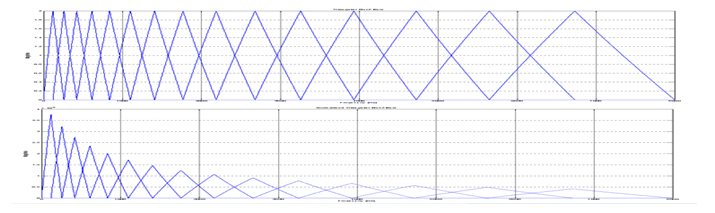Inverse DFT – The IDFT of the Mel spectrum is computed, resulting in the cepstrum. This representation is valuable because it separates characteristics of the source and vocal tract from the speech waveform. The first 12 values of the resulting cepstrum are recorded.

Additional Features -

• Energy Feature – This step is performed in parallel with the MFCC feature extraction and involves calculating the total energy of the input frame.

• Delta MFCC Features – In order to capture the changes in speech from frame-to-frame, the first and second derivative of the MFCC coefficients are also calculated and included.

The LPC (Linear Predictive Coding) smoothed MFCCs, and Enhanced MFCCs are described in (Këpuska & Klein, 2009). Those triple features are used by the Back End to score each with its corresponding HMM model.

2. VAD CLASSIFICATION

In the first phase, for every input frame VAD decides whether the frame is speech-like or non-speech-like. Several methods have been implemented and tested to solve this problem.

In the first implementation, the decision was made based on three features: log energy difference, spectral difference, and MFCC difference. A threshold was determined empirically for each feature, and the frame was considered speech-like if at least two out of the three features were above the threshold. This was in effect a Decision Tree classifier, and the decision regions consisted of hypercubes in the feature space.

In order to improve the VAD classification accuracy, research has been carried out to determine the ideal features to be used for classification. Hence, Artificial Neural Networks (ANN) and Support Vector Machines (SVM) were tested for automatic classification. One attempt was to take several important features from a stream of consecutive frames and classify them using ANN or SVM. The idea was that the classifier would make a better decision if shown multiple consecutive frames rather than a single frame. The result, although good, was too computationally expensive, and the final implementation still uses information from only a single frame.

#### 2.1. FIRST VAD PHASE – SINGLE FRAME DECISION

The final implementation uses the same three features as in the first implementation: log energy difference, spectral difference, and MFCC difference; however, classification is performed using a linear SVM. There are several advantages over the original method. First, the classification boundary in the feature space is a hyperplane, which is more robust than the hypercubes produced by the decision tree method. Second, the thresholds do not have to be picked manually but can be trained automatically (and optimally) using marked input files. Third, the sensitivity can be adjusted in smooth increments using a single parameter, the SVM decision threshold. Recall that the output of a SVM is a single scalar, u=wxbu=w⋅x−b(Klein, 2007). Usually the decision threshold is set at u=0, but it can be adjusted in either direction depending on the requirements. Finally, the linear SVM kernel is extremely efficient, because classification of new data requires just a single dot product computation.

The following figures show the training data scattered on two dimensional planes, followed by a 3 dimensional representation which includes the SVM separating plane.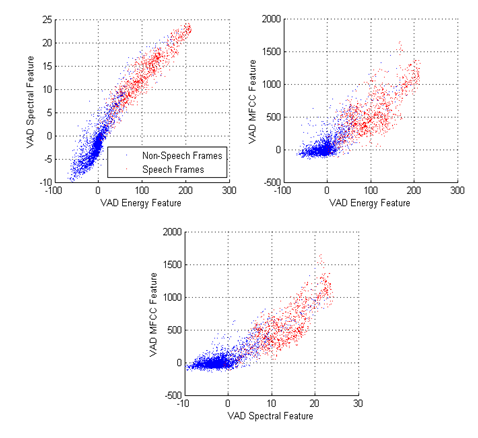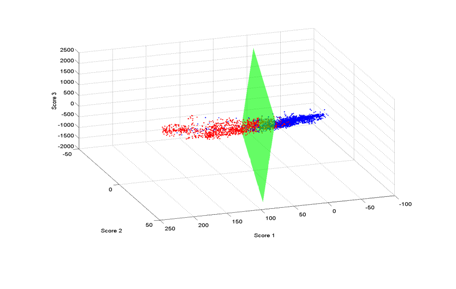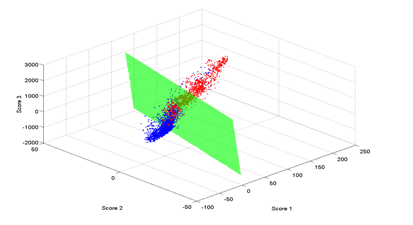In the figures above, the red points correspond to speech frames while the blue points correspond to non-speech frames, as labeled by a human listener. It can be seen that the linear classifier produces a fairly good separating plane between the two classes, and the plane could be moved in either direction by adjusting the threshold.

#### 2.2. SECOND VAD PHASE – FINAL DECISION LOGIC

In the second phase, the VAD keeps track of the number of frames marked as speech and non-speech and makes a final decision. There are four parameters: MIN_VAD_ON_COUNT, MIN_VAD_OFF_COUNT, LEAD_COUNT, and TRAIL_COUNT. The algorithm calls for a number of consecutive frames to be marked as speech in order to set the state to VAD_ON; this number is specified by MIN_VAD_ON_COUNT. It also requires a number of consecutive frames to be marked as non-speech in order to set the state to VAD_OFF; this number is specified by MIN_VAD_OFF_COUNT. Because the classifier can make mistakes at the beginning and the end, the logic also includes a lead-in and a trail-out time. When the minimum number of consecutive speech frames has been observed, VAD does not indicate VAD_ON for the first of those frames. Rather it selects the frame that was observed a number time instances earlier; this number is specified by LEAD_COUNT. Similarly, when the minimum number of non-speech frames has been observed, VAD waits an additional number of frames before changing to VAD_OFF, specified by TRAIL_COUNT.

### 3.BACK END - PLAIN HMM SCORES

The Back End is responsible for scoring observation sequences. The WUW-SR system uses a Hidden Markov Models for acoustic modeling, and as a result the back end consists of a HMM recognizer. Prior to recognition, HMM model(s) must be created and trained for the word or phrase which is selected to be the Wake-Up-Word.

When the VAD state changes from VAD_OFF to VAD_ON, the HMM recognizer resets and prepares for a new observation sequence. As long as the VAD state remains VAD_ON, feature vectors are continuously passed to the HMM recognizer, where they are scored using the novel triple scoring method. If using multiple feature streams, recognition is performed for each stream in parallel. When VAD state changes from VAD_ON to VAD_OFF, multiple scores (e.g., MFCC, LPC and E-MFCC Score) are obtained from the HMM recognizer and are sent to the SVM classifier. SVM produces a classification score which is compared against a threshold to make the final classification decision of INV or OOV.

For the first tests on speech data, a HMM was trained on the word “operator.” The training sequences were taken from the CCW17 and WUW-II (Këpuska & Klein, 2009) corpora for a total of 573 sequences from over 200 different speakers. After features were extracted, some of the erroneous VAD segments were manually removed. The INV testing sequences were the same as the training sequences, while the OOV testing sequences included the rest of the CCW17 corpus (3833 utterances, 9 different words, over 200 different speakers). The HMM was a left-to-right model with no skips, 30 states, and 6 mixtures per state, and was trained with two iterations of Baum-Welch.

The score is the result of the Viterbi algorithm over the input sequence. Recall that the Viterbi algorithm finds the state sequence that has the highest probability of being taken while generating the observation sequence. The final score is that probability normalized by the number of input observations, T. The Figure 8 below shows the result:

The distributions look Gaussian, but there is significant overlap between them. The equal error rate of 15.5% essentially means that at that threshold, 15.5% of the OOV words would be classified as INV, and 15.5% of the INV words would be classified as OOV. Obviously, no practical applications can be developed based on the performance of this recognizer.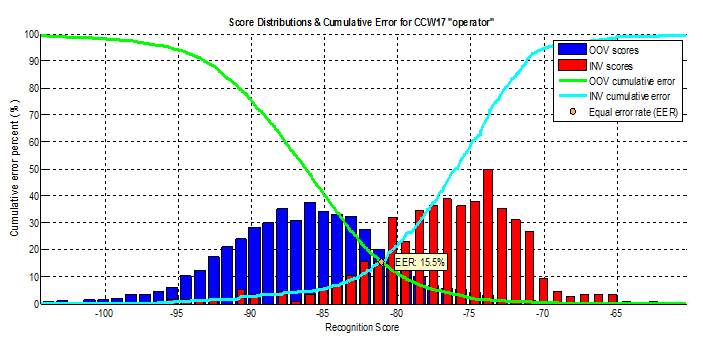### 4. SVM CLASSIFICATION

After HMM recognition, the algorithm uses two additional scores for any given observation sequence (e.g., MFCC, LPC and e-MFCC). When considering the three scores as features in a three dimensional space, the separation between INV and OOV distributions increases significantly. The next experiment runs recognition on the same data as above, but this time the recognizer uses the triple scoring algorithm to output three scores (Këpuska & Klein, 2009).

#### 4.1. TRIPLE SCORING METHOD

The figures below show two-dimensional scatter plots of Score 1 vs. Score 2, and Score 1 vs. Score 3 for each observation sequence (e.g., MFCC, LPC and e-MFCC). In addition, a histogram on the horizontal axis shows the distributions of Score 1 independently, and a similar histogram on the vertical axis shows the distributions of Score 2 and Score 3 independently. The histograms are hollowed out so that the overlap between distributions can be seen clearly. The distribution for Score 1 is exactly the same as in the previous section, as the data and model haven’t changed. Any individual score does not produce a good separation between classes, and in fact the Score 2 distributions have almost complete overlap. However, the two dimensional separation in either case is remarkable. When all three scores are considered in a three dimensional space, their separation is even better than either two dimensions as depicted in Figure12 and Figure 13.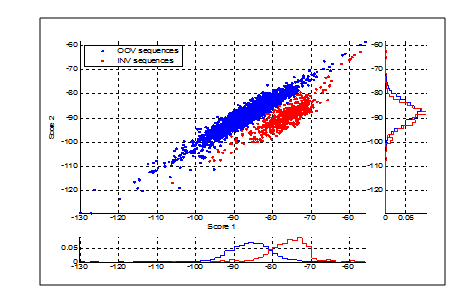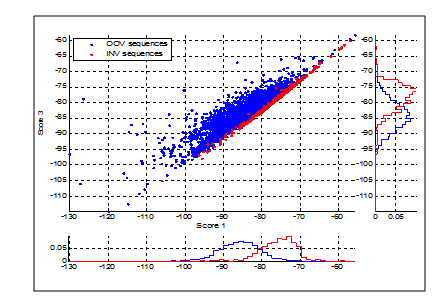In order to automatically classify an input sequence as INV or OOV, the triple score feature space,3ℛ3,can be partitioned by a binary classifier into two regions,31ℛ13and 31ℛ−13. The SVMs have been selected for this task because of the following reasons: they can produce various kinds of decision surfaces including radial basis function, polynomial, and linear; they employ Structural Risk Minimization (SRM) (Burges, 1998) to maximize the margin which has shown empirically to have good generalization performance.

#### 4.2. SVM PARAMETERS

Two types of SVMs have been considered for this task: linear and RBF. The linear SVM uses a dot product kernel function, K(x,y)=xyK(x,y)=x⋅y, and separates the feature space with a hyperplane. It is very computationally efficient because no matter how many support vectors are found, evaluation requires only a single dot product. Figure 14above shows that the separation between distributions based on Score 1 and Score 3 is almost linear, so a linear SVM would likely give good results. However, in the Score 1/Score 2 space, the distributions have a curvature, so the linear SVM is unlikely to generalize well for unseen data. The figures below show the decision boundary found by a linear SVM trained on Score 1+2, and Score 1+3, respectively.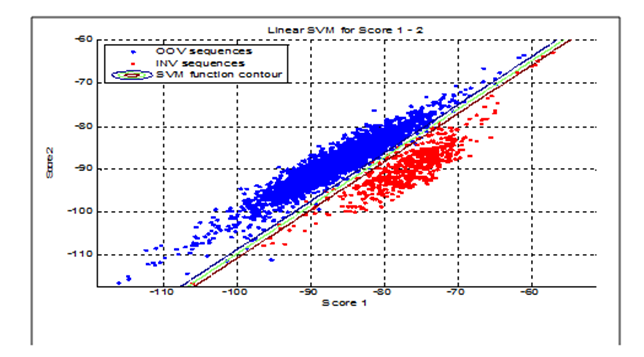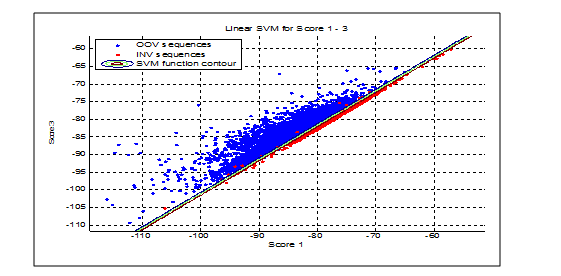The line in the center represents the contour of the SVM function at u=0, and outer two lines are drawn at u=±1u=±1. Using 0 as the threshold, the accuracy of Scores 1 - 2 is 99.7% Correct Rejection (CR) and 98.6% Correct Acceptance (CA), while for Scores 1 – 3 it is 99.5% CR and 95.5% CA. If considering only two features, Scores 1 and 2 seem to have better classification ability. However, combining the three scores produces the plane shown below from two different angles.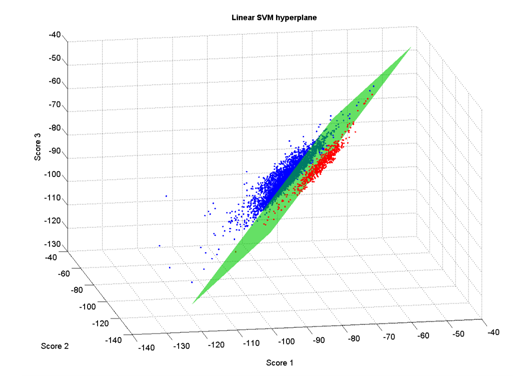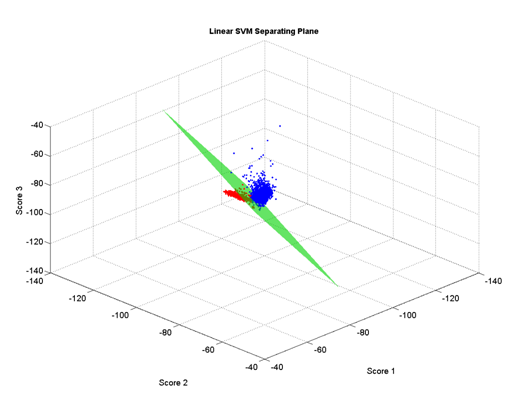The plane split the feature space with an accuracy of 99.9% CR and 99.5% CA (just 6 of 4499 total sequences were misclassified). The accuracy was better than any of the 2 dimensional cases, indicating that Score 3 contains additional information not found in Score 2. The classification error rate of the linear SVM is shown below: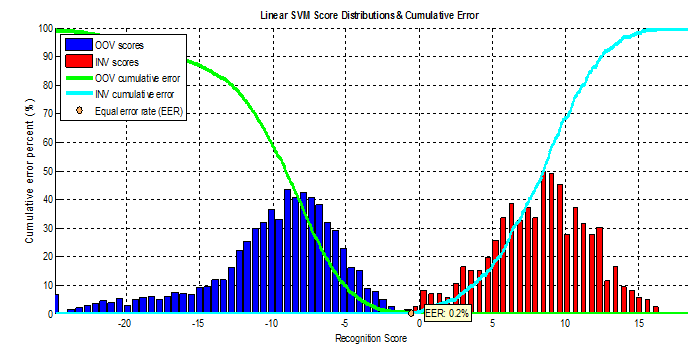The conclusion is that using the triple scoring method combined with a linear SVM decreased the equal error rate on this particular data set from 15.5% to 0.2%, or in other words increased accuracy by over 76.5 times (i.e., error rate reduction of 7650%)!

In the next experiment, a Radial Basis Function (RBF) kernel was used for the SVM. The RBF function, K(x,y)=eγxy2K(x,y)=e−γ|x−y|2, maps feature vectors into an infinitely dimensional Hilbert space and is able to achieve complete separation between classes in most cases. However, the γγ parameter must be chosen carefully in order to avoid overtraining. As there is no way to determine it automatically, a grid search may be used to find a good value. For most experiments γ=0.008γ=0.008 gave good results. Shown below are the RBF SVM contours for both two-dimensional cases.